# Selina Solutions Concise Maths Class 10 Chapter 15 Similarity (With Applications to Maps and Models) Exercise 15(A)

Problems on proving similarity of triangles using any one of the conditions (SAS, AA or AAA and SSS) and later by corresponding sides and corresponding angles proving the required are covered in this exercise. Students wanting any help or guidance in solving problems can make use of the Selina Solutions for Class 10 Maths. The solutions are prepared by subject matter experts at BYJU’S. For more conceptual knowledge, students can access Selina Solutions Concise Maths Class 10 Chapter 15 Similarity (With Applications to Maps and Models) Exercise 15(A) PDF, provided in the links below.

## Selina Solutions Concise Maths Class 10 Chapter 15 Similarity (With Applications to Maps and Models) Exercise 15(A) Download PDF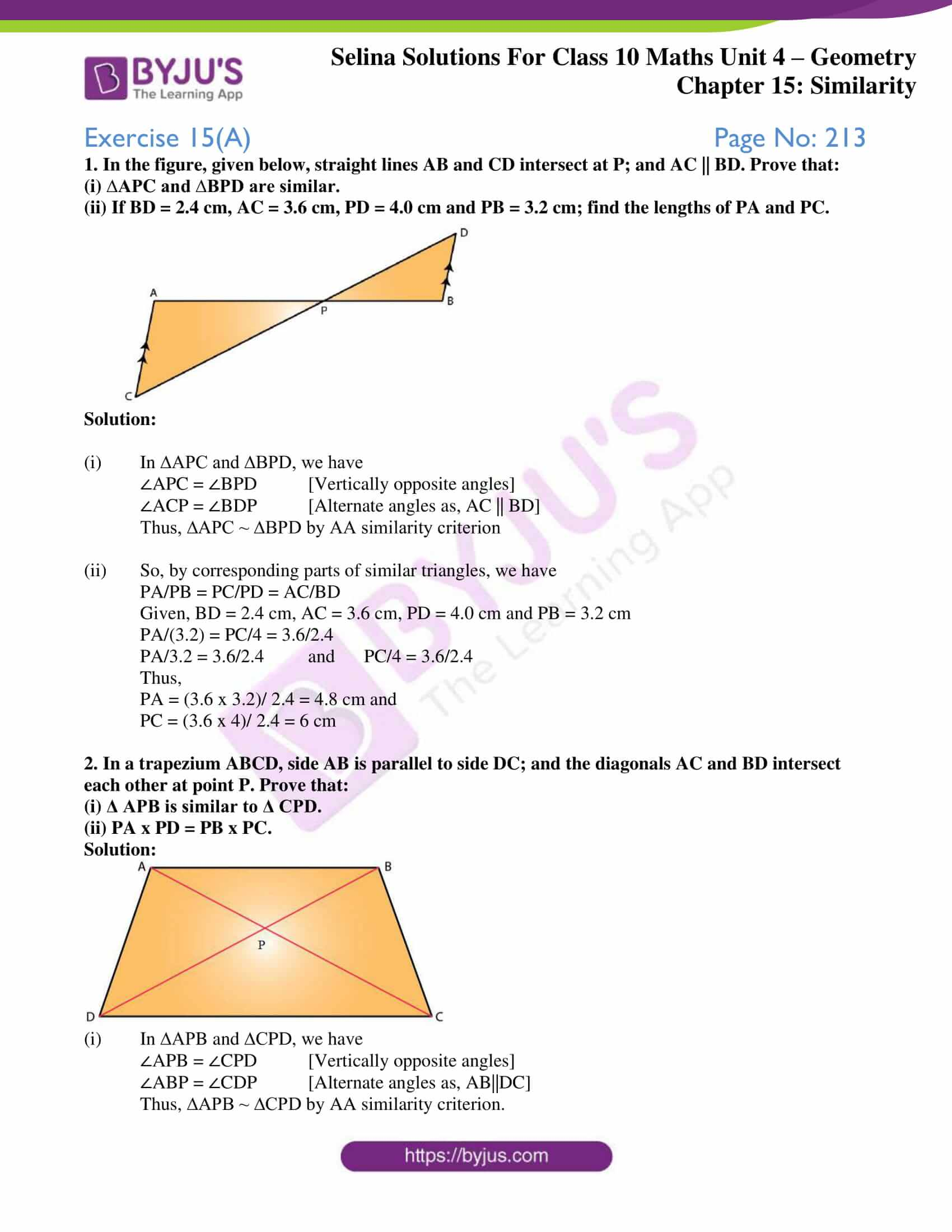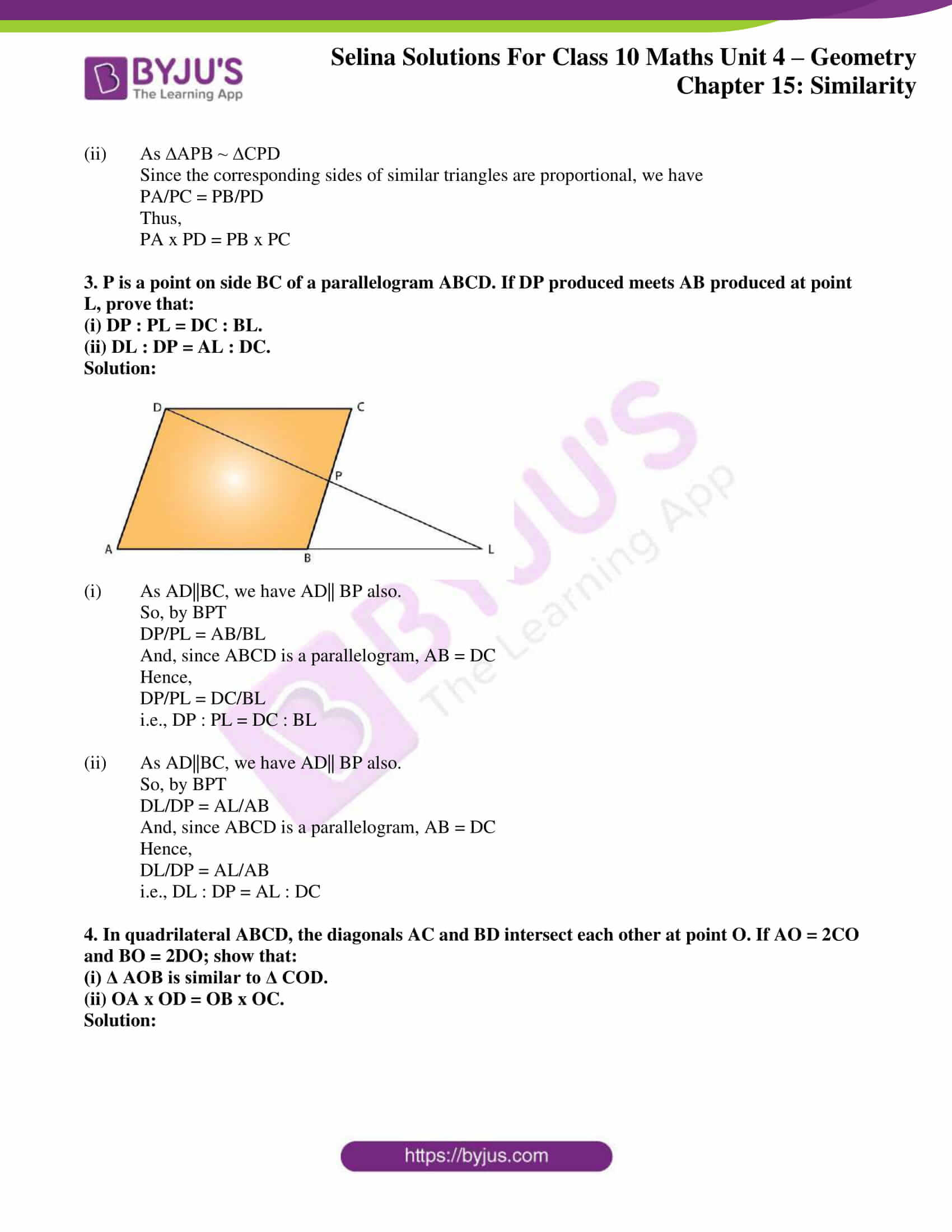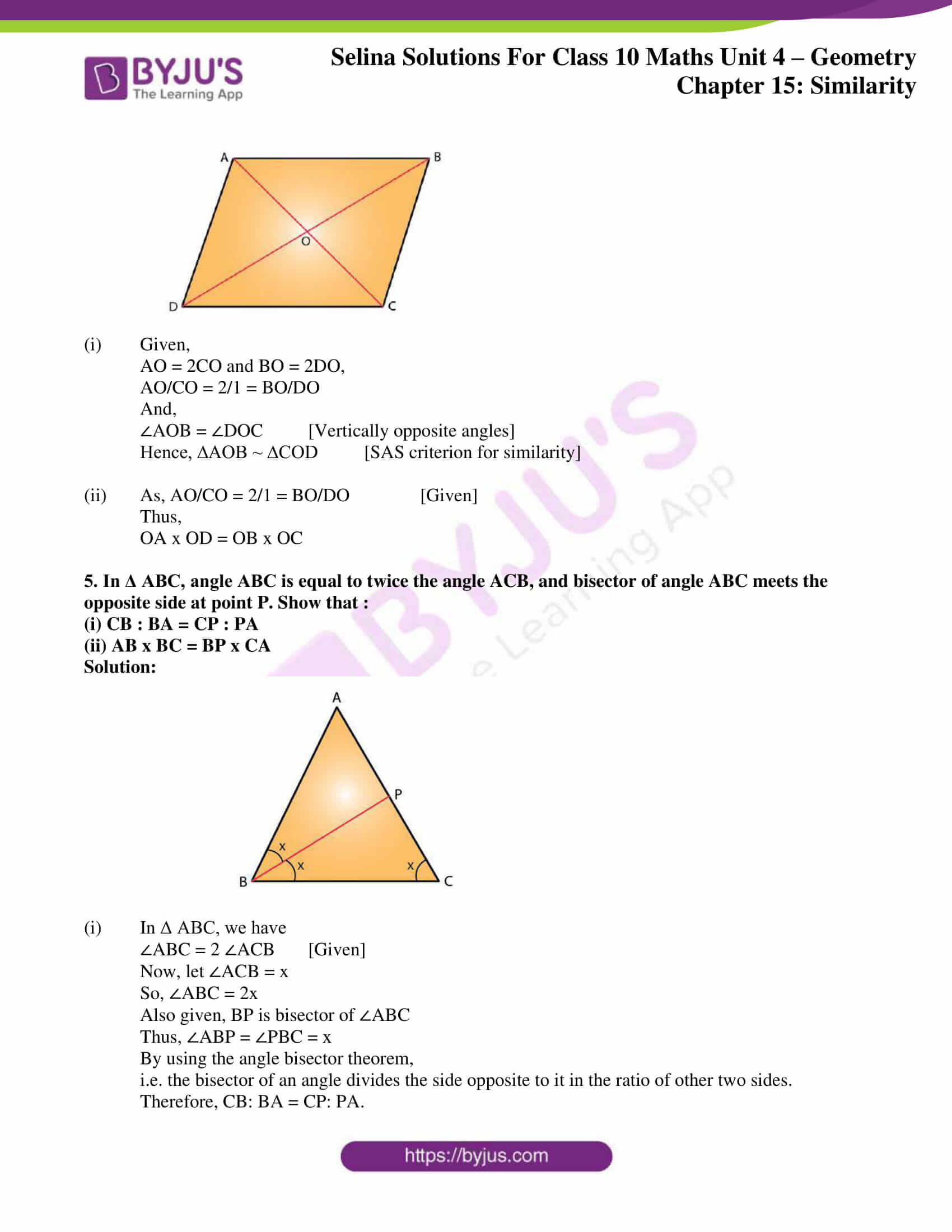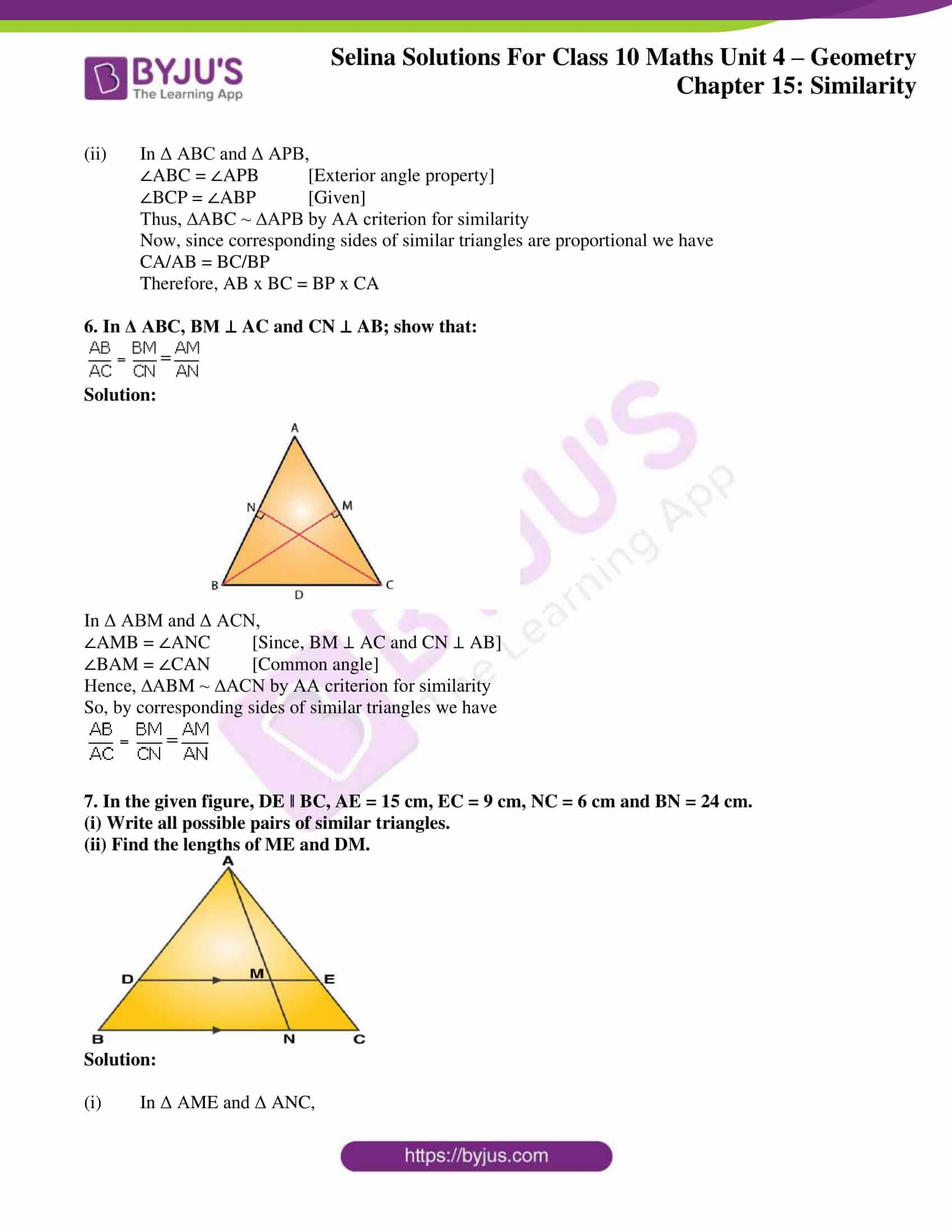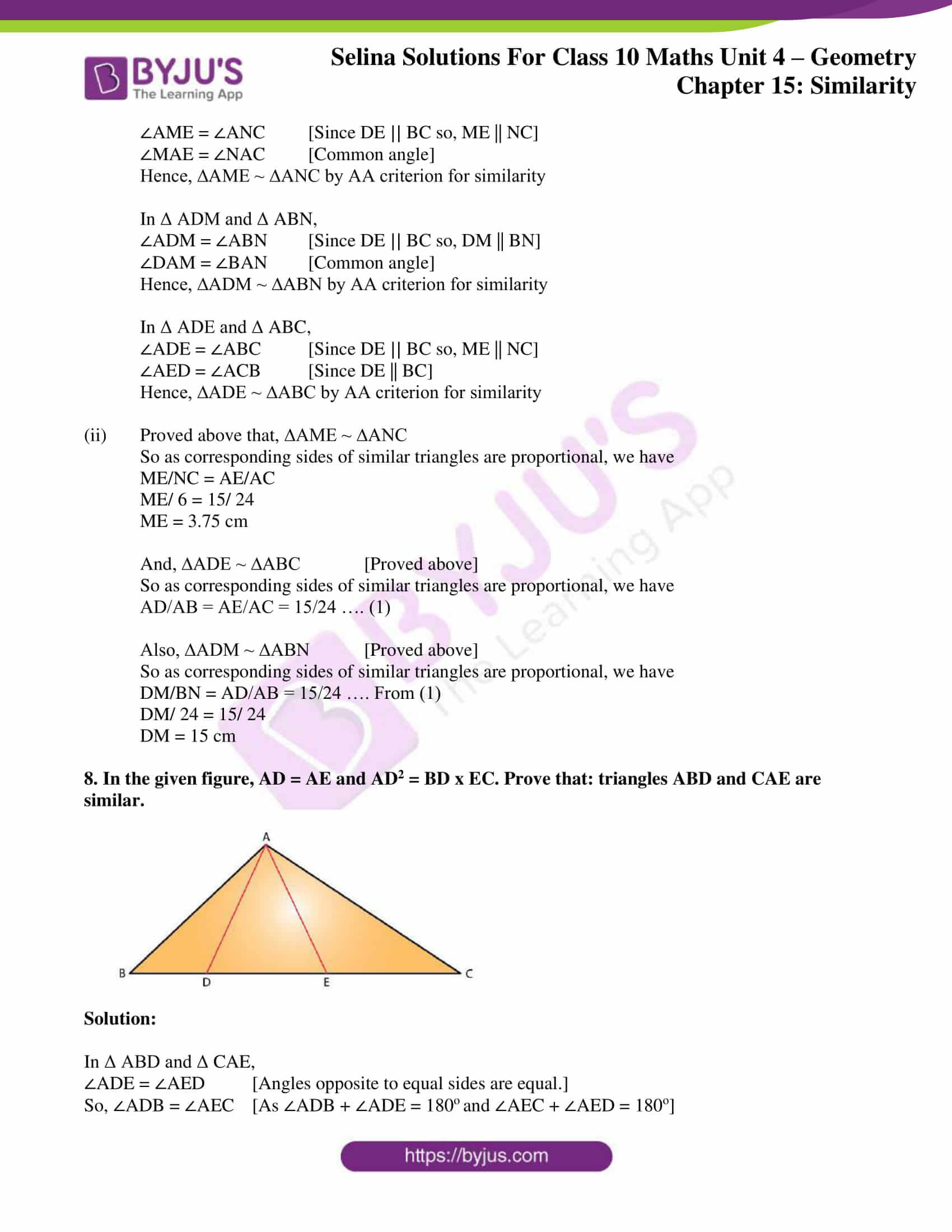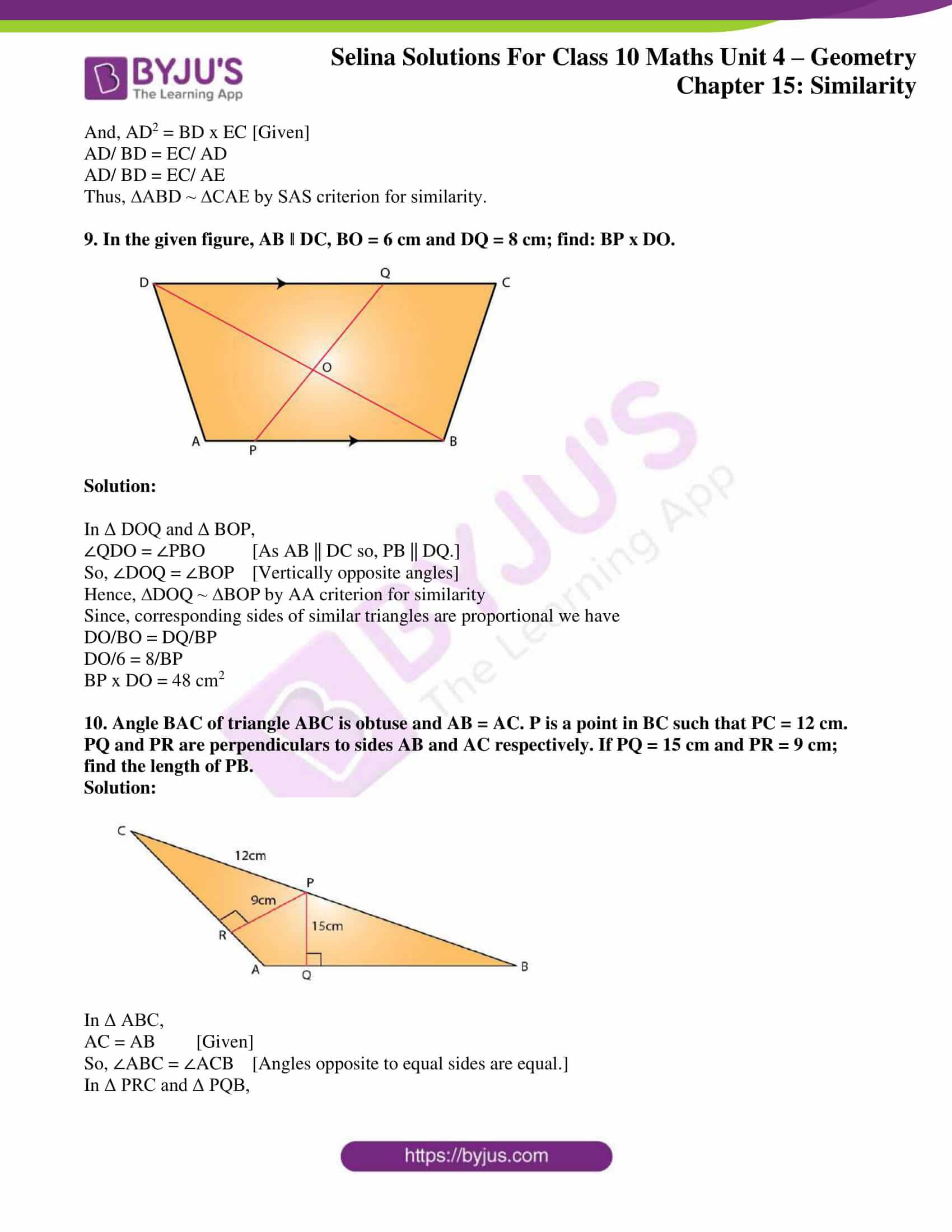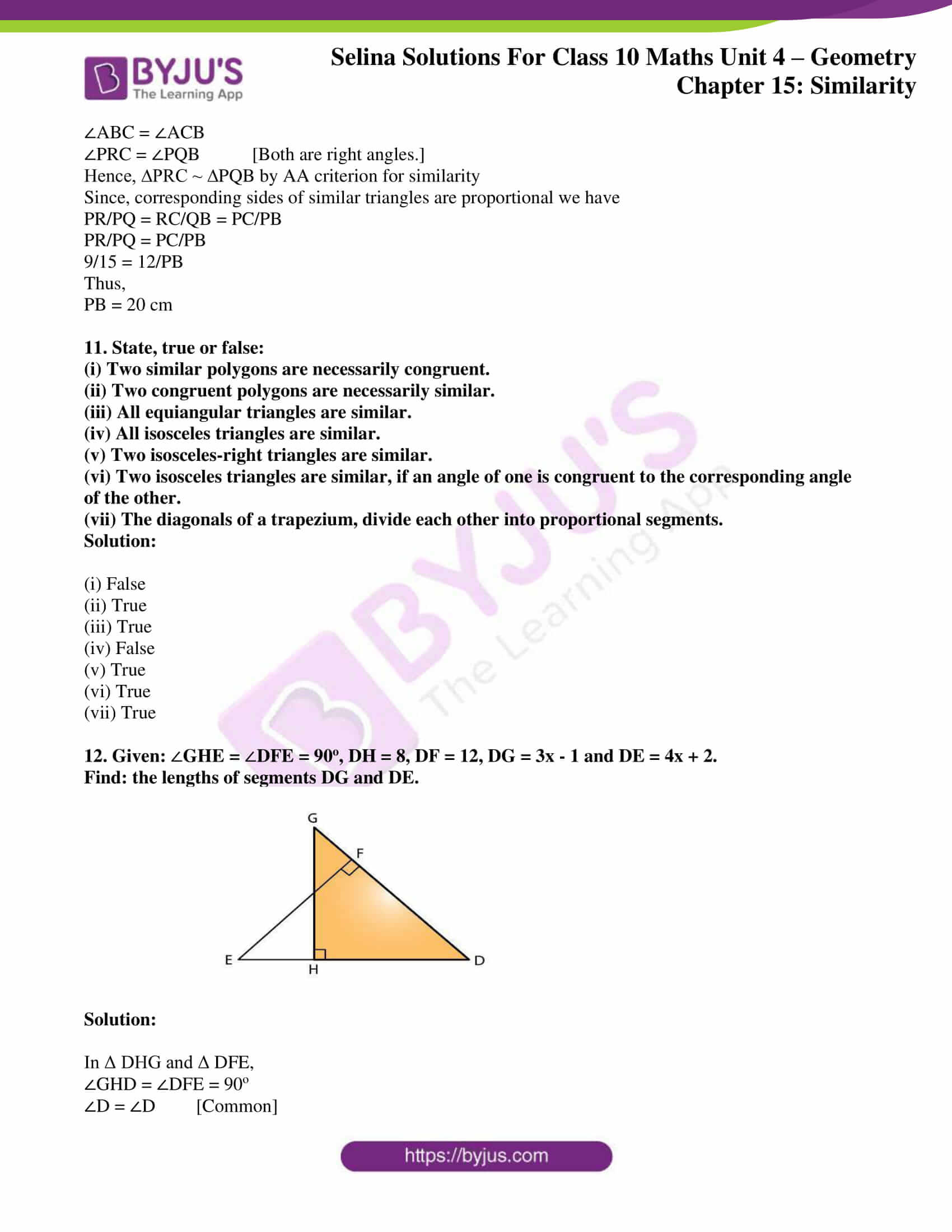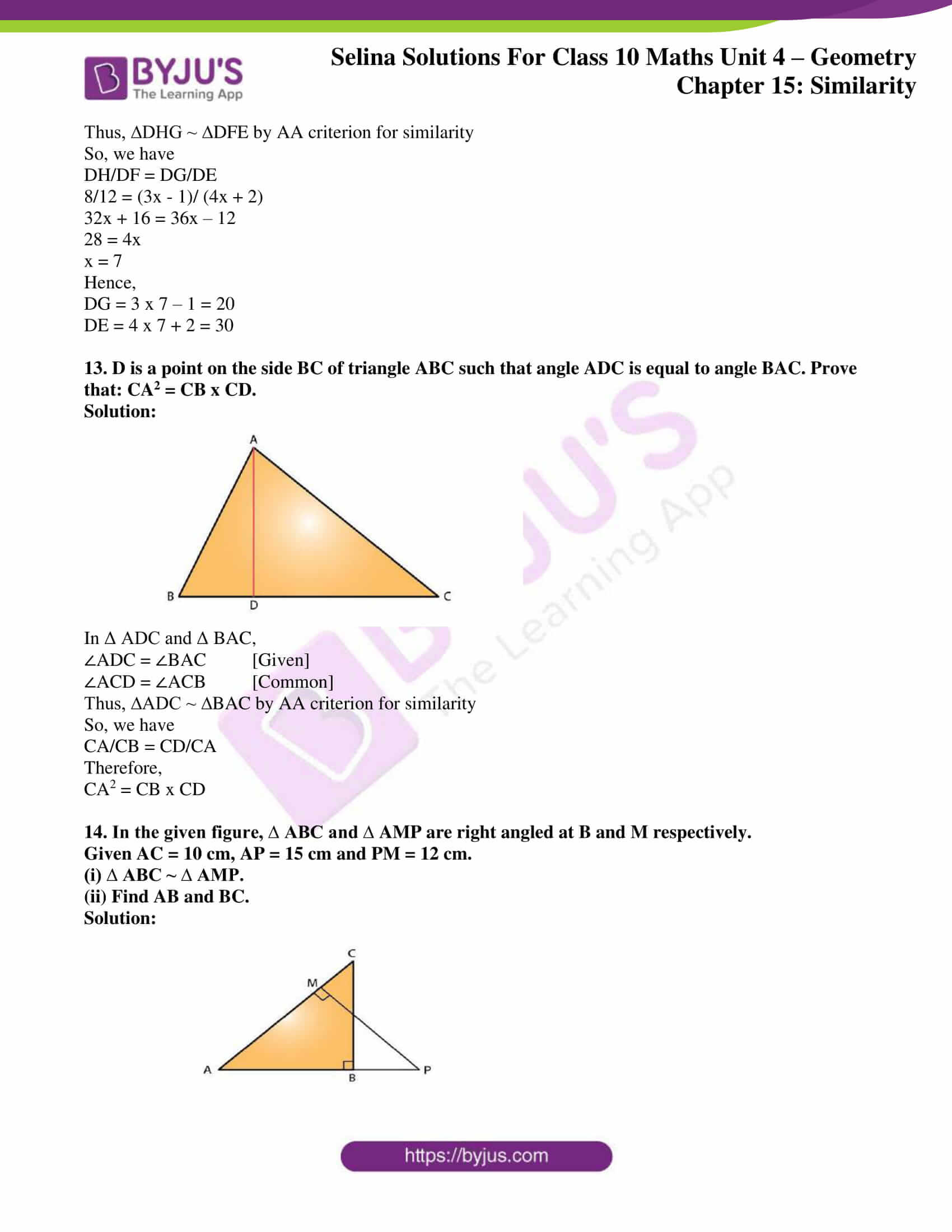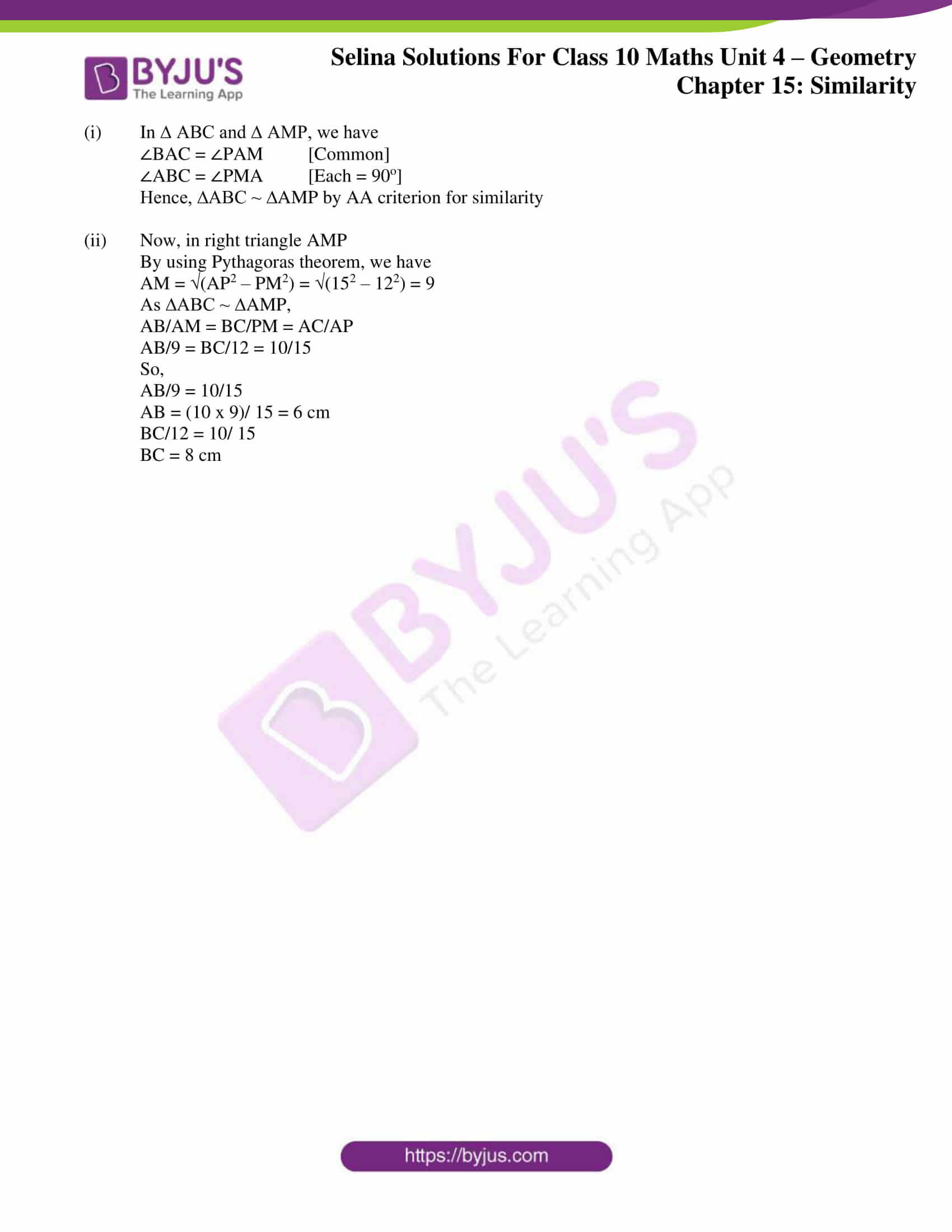## Access Selina Solutions Concise Maths Class 10 Chapter 15 Similarity (With Applications to Maps and Models)

#### Exercise 15(A) Page No: 213

1. In the figure, given below, straight lines AB and CD intersect at P; and AC || BD. Prove that:

(i) ∆APC and ∆BPD are similar.

(ii) If BD = 2.4 cm, AC = 3.6 cm, PD = 4.0 cm and PB = 3.2 cm; find the lengths of PA and PC.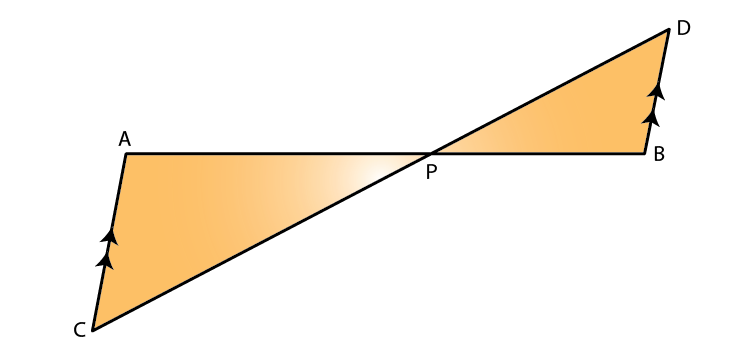Solution:

(i) In ∆APC and ∆BPD, we have

∠APC = ∠BPD [Vertically opposite angles]

∠ACP = ∠BDP [Alternate angles as, AC || BD]

Thus, ∆APC ~ ∆BPD by AA similarity criterion

(ii) So, by corresponding parts of similar triangles, we have

PA/PB = PC/PD = AC/BD

Given, BD = 2.4 cm, AC = 3.6 cm, PD = 4.0 cm and PB = 3.2 cm

PA/(3.2) = PC/4 = 3.6/2.4

PA/3.2 = 3.6/2.4 and PC/4 = 3.6/2.4

Thus,

PA = (3.6 x 3.2)/ 2.4 = 4.8 cm and

PC = (3.6 x 4)/ 2.4 = 6 cm

2. In a trapezium ABCD, side AB is parallel to side DC; and the diagonals AC and BD intersect each other at point P. Prove that:

(i) Δ APB is similar to Δ CPD.

(ii) PA x PD = PB x PC.

Solution: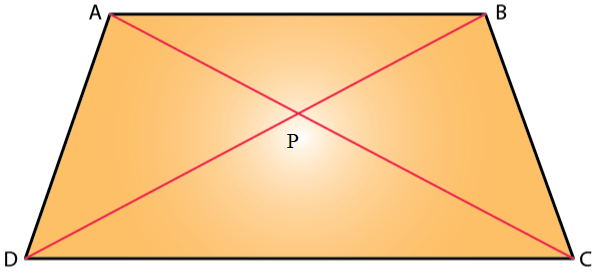(i) In ∆APB and ∆CPD, we have

∠APB = ∠CPD [Vertically opposite angles]

∠ABP = ∠CDP [Alternate angles as, AB||DC]

Thus, ∆APB ~ ∆CPD by AA similarity criterion.

(ii) As ∆APB ~ ∆CPD

Since the corresponding sides of similar triangles are proportional, we have

PA/PC = PB/PD

Thus,

PA x PD = PB x PC

3. P is a point on side BC of a parallelogram ABCD. If DP produced meets AB produced at point L, prove that:

(i) DP : PL = DC : BL.

(ii) DL : DP = AL : DC.

Solution: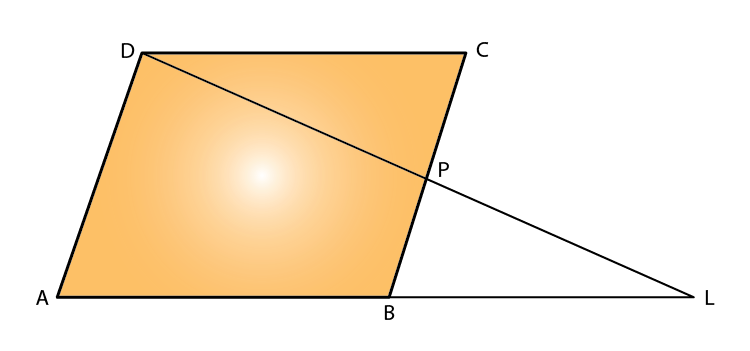So, by BPT

DP/PL = AB/BL

And, since ABCD is a parallelogram, AB = DC

Hence,

DP/PL = DC/BL

i.e., DP : PL = DC : BL

So, by BPT

DL/DP = AL/AB

And, since ABCD is a parallelogram, AB = DC

Hence,

DL/DP = AL/AB

i.e., DL : DP = AL : DC

4. In quadrilateral ABCD, the diagonals AC and BD intersect each other at point O. If AO = 2CO and BO = 2DO; show that:

(i) Δ AOB is similar to Δ COD.

(ii) OA x OD = OB x OC.

Solution: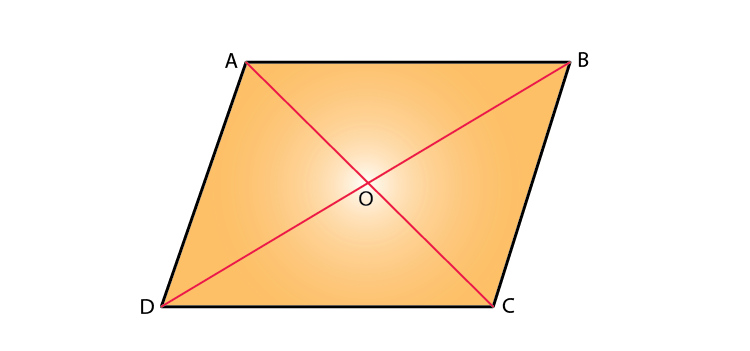(i) Given,

AO = 2CO and BO = 2DO,

AO/CO = 2/1 = BO/DO

And,

∠AOB = ∠DOC [Vertically opposite angles]

Hence, ∆AOB ~ ∆COD [SAS criterion for similarity]

(ii) As, AO/CO = 2/1 = BO/DO [Given]

Thus,

OA x OD = OB x OC

5. In Δ ABC, angle ABC is equal to twice the angle ACB, and bisector of angle ABC meets the opposite side at point P. Show that :

(i) CB : BA = CP : PA

(ii) AB x BC = BP x CA

Solution: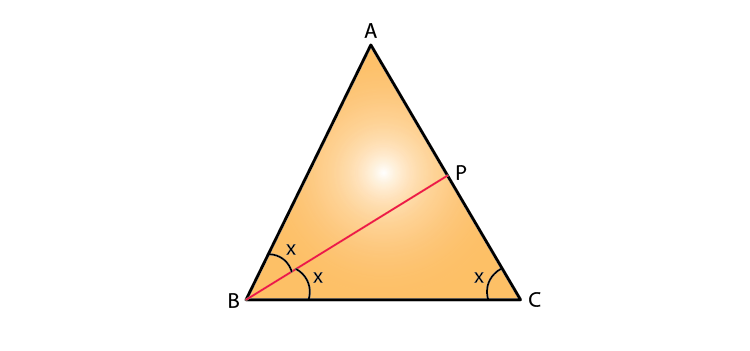(i) In Δ ABC, we have

∠ABC = 2 ∠ACB [Given]

Now, let ∠ACB = x

So, ∠ABC = 2x

Also given, BP is bisector of ∠ABC

Thus, ∠ABP = ∠PBC = x

By using the angle bisector theorem,

i.e. the bisector of an angle divides the side opposite to it in the ratio of other two sides.

Therefore, CB: BA = CP: PA.

(ii) In Δ ABC and Δ APB,

∠ABC = ∠APB [Exterior angle property]

∠BCP = ∠ABP [Given]

Thus, ∆ABC ~ ∆APB by AA criterion for similarity

Now, since corresponding sides of similar triangles are proportional we have

CA/AB = BC/BP

Therefore, AB x BC = BP x CA

6. In Δ ABC, BM ⊥ AC and CN ⊥ AB; show that: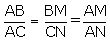Solution: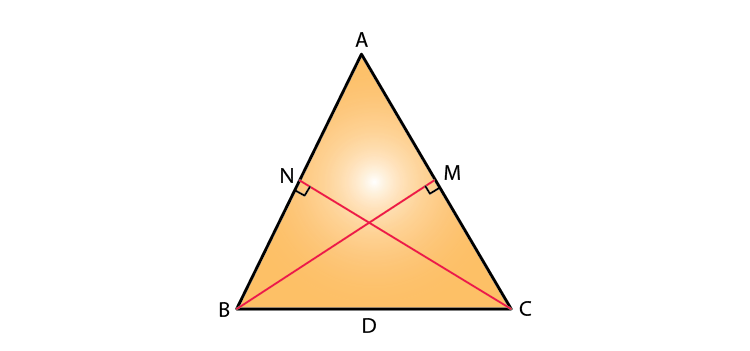In Δ ABM and Δ ACN,

∠AMB = ∠ANC [Since, BM ⊥ AC and CN ⊥ AB]

∠BAM = ∠CAN [Common angle]

Hence, ∆ABM ~ ∆ACN by AA criterion for similarity

So, by corresponding sides of similar triangles we have7. In the given figure, DE ‖ BC, AE = 15 cm, EC = 9 cm, NC = 6 cm and BN = 24 cm.

(i) Write all possible pairs of similar triangles.

(ii) Find the lengths of ME and DM.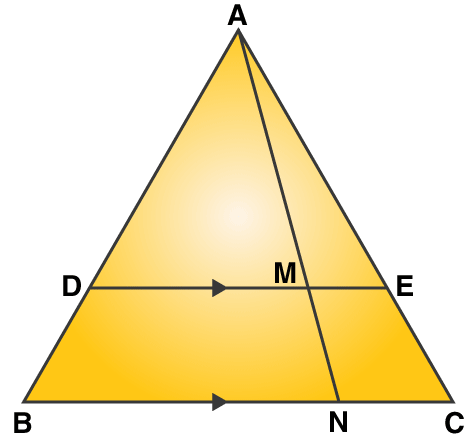Solution:

(i) In Δ AME and Δ ANC,

∠AME = ∠ANC [Since DE || BC so, ME || NC]

∠MAE = ∠NAC [Common angle]

Hence, ∆AME ~ ∆ANC by AA criterion for similarity

In Δ ADM and Δ ABN,

∠ADM = ∠ABN [Since DE || BC so, DM || BN]

∠DAM = ∠BAN [Common angle]

Hence, ∆ADM ~ ∆ABN by AA criterion for similarity

In Δ ADE and Δ ABC,

∠ADE = ∠ABC [Since DE || BC so, ME || NC]

∠AED = ∠ACB [Since DE || BC]

Hence, ∆ADE ~ ∆ABC by AA criterion for similarity

(ii) Proved above that, ∆AME ~ ∆ANC

So as corresponding sides of similar triangles are proportional, we have

ME/NC = AE/AC

ME/ 6 = 15/ 24

ME = 3.75 cm

And, ∆ADE ~ ∆ABC [Proved above]

So as corresponding sides of similar triangles are proportional, we have

AD/AB = AE/AC = 15/24 …. (1)

Also, ∆ADM ~ ∆ABN [Proved above]

So as corresponding sides of similar triangles are proportional, we have

DM/BN = AD/AB = 15/24 …. From (1)

DM/ 24 = 15/ 24

DM = 15 cm

8. In the given figure, AD = AE and AD2 = BD x EC. Prove that: triangles ABD and CAE are similar.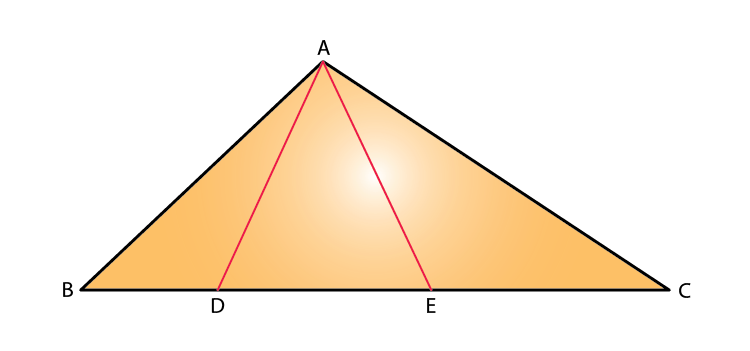Solution:

In Δ ABD and Δ CAE,

∠ADE = ∠AED [Angles opposite to equal sides are equal.]

And, AD2 = BD x EC [Given]

Thus, ∆ABD ~ ∆CAE by SAS criterion for similarity.

9. In the given figure, AB ‖ DC, BO = 6 cm and DQ = 8 cm; find: BP x DO.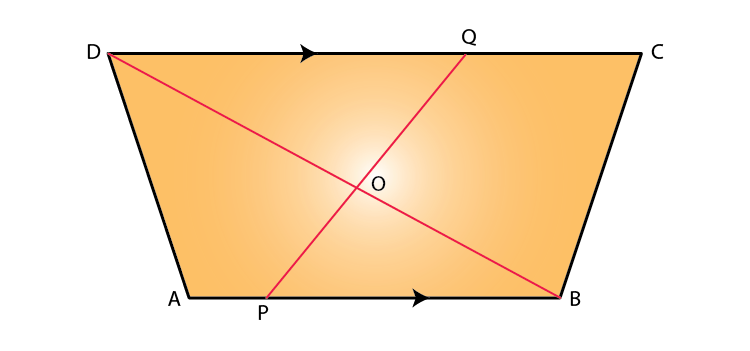Solution:

In Δ DOQ and Δ BOP,

∠QDO = ∠PBO [As AB || DC so, PB || DQ.]

So, ∠DOQ = ∠BOP [Vertically opposite angles]

Hence, ∆DOQ ~ ∆BOP by AA criterion for similarity

Since, corresponding sides of similar triangles are proportional we have

DO/BO = DQ/BP

DO/6 = 8/BP

BP x DO = 48 cm2

10. Angle BAC of triangle ABC is obtuse and AB = AC. P is a point in BC such that PC = 12 cm. PQ and PR are perpendiculars to sides AB and AC respectively. If PQ = 15 cm and PR = 9 cm; find the length of PB.

Solution: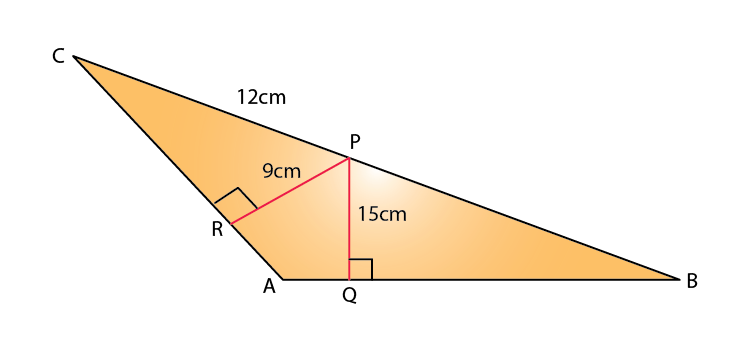In Δ ABC,

AC = AB [Given]

So, ∠ABC = ∠ACB [Angles opposite to equal sides are equal.]

In Δ PRC and Δ PQB,

∠ABC = ∠ACB

∠PRC = ∠PQB [Both are right angles.]

Hence, ∆PRC ~ ∆PQB by AA criterion for similarity

Since, corresponding sides of similar triangles are proportional we have

PR/PQ = RC/QB = PC/PB

PR/PQ = PC/PB

9/15 = 12/PB

Thus,

PB = 20 cm

11. State, true or false:

(i) Two similar polygons are necessarily congruent.

(ii) Two congruent polygons are necessarily similar.

(iii) All equiangular triangles are similar.

(iv) All isosceles triangles are similar.

(v) Two isosceles-right triangles are similar.

(vi) Two isosceles triangles are similar, if an angle of one is congruent to the corresponding angle of the other.

(vii) The diagonals of a trapezium, divide each other into proportional segments.

Solution:

(i) False

(ii) True

(iii) True

(iv) False

(v) True

(vi) True

(vii) True

12. Given: GHE = DFE = 90o, DH = 8, DF = 12, DG = 3x – 1 and DE = 4x + 2.

Find: the lengths of segments DG and DE.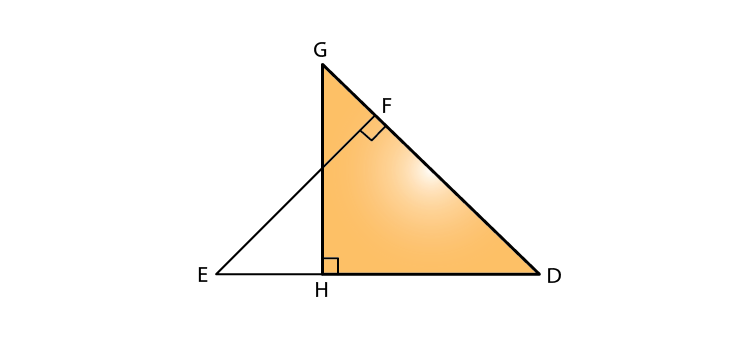Solution:

In Δ DHG and Δ DFE,

∠GHD = ∠DFE = 90o

∠D = ∠D [Common]

Thus, ∆DHG ~ ∆DFE by AA criterion for similarity

So, we have

DH/DF = DG/DE

8/12 = (3x – 1)/ (4x + 2)

32x + 16 = 36x – 12

28 = 4x

x = 7

Hence,

DG = 3 x 7 – 1 = 20

DE = 4 x 7 + 2 = 30

13. D is a point on the side BC of triangle ABC such that angle ADC is equal to angle BAC. Prove that: CA2 = CB x CD.

Solution: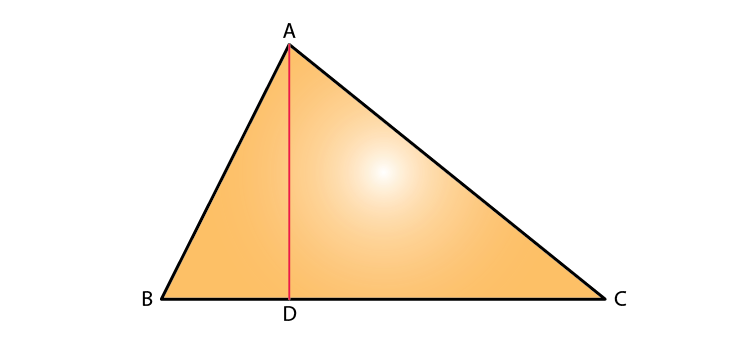In Δ ADC and Δ BAC,

∠ACD = ∠ACB [Common]

Thus, ∆ADC ~ ∆BAC by AA criterion for similarity

So, we have

CA/CB = CD/CA

Therefore,

CA2 = CB x CD

14. In the given figure, ∆ ABC and ∆ AMP are right angled at B and M respectively.

Given AC = 10 cm, AP = 15 cm and PM = 12 cm.

(i) ∆ ABC ~ ∆ AMP.

(ii) Find AB and BC.

Solution: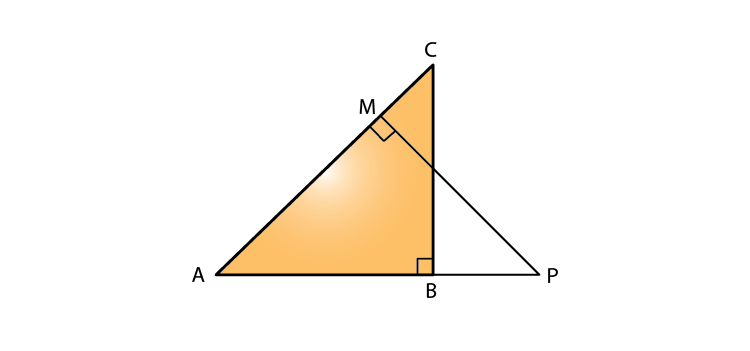(i) In ∆ ABC and ∆ AMP, we have

∠BAC = ∠PAM [Common]

∠ABC = ∠PMA [Each = 90o]

Hence, ∆ABC ~ ∆AMP by AA criterion for similarity

(ii) Now, in right triangle AMP

By using Pythagoras theorem, we have

AM = √(AP2 – PM2) = √(152 – 122) = 9

As ∆ABC ~ ∆AMP,

AB/AM = BC/PM = AC/AP

AB/9 = BC/12 = 10/15

So,

AB/9 = 10/15

AB = (10 x 9)/ 15 = 6 cm

BC/12 = 10/ 15

BC = 8 cm

### Access other exercises of Selina Solutions Concise Maths Class 10 Chapter 15 Similarity (With Applications to Maps and Models)

Exercise 15(B) Solutions

Exercise 15(C) Solutions

Exercise 15(D) Solutions

Exercise 15(E) Solutions